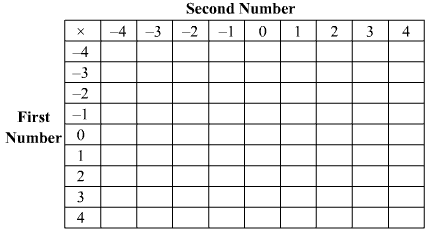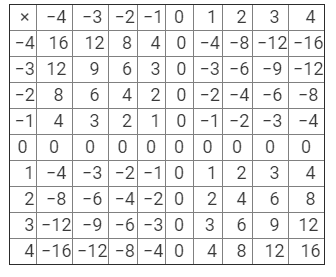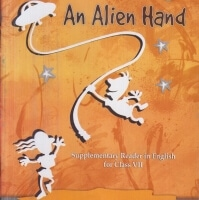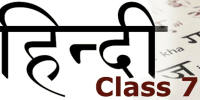Courses

# RD Sharma Solutions - Ex-1.1 Integers, Class 7, Math Class 7 Notes | EduRev

## Class 7: RD Sharma Solutions - Ex-1.1 Integers, Class 7, Math Class 7 Notes | EduRev

The document RD Sharma Solutions - Ex-1.1 Integers, Class 7, Math Class 7 Notes | EduRev is a part of the Class 7 Course RD Sharma Solutions for Class 7 Mathematics.
All you need of Class 7 at this link: Class 7

Q 1. Determine each of the following products:
(i) 12 ☓ 7
(ii) (−15) ☓ 8
(iii) (−25) ☓ (−9)
(iv) 125 ☓ (−8)

(i) 12 × 7 = 84
(ii) (−15) × 8 =  −120
(iii) (−25) × (−9) =  225
(iv) 125 × (−8) =  −1000

Q 2. Find each of the following products:
(i) 3 ☓ (−8) ☓ 5
(ii) 9 ☓ (−3) ☓ (−6)
(iii) (−2) ☓ 36 ☓ (−5)
(iv) (−2) ☓ (−4) ☓ (−6) ☓ (−8)

(i) 3 × (−8) × 5 = −3 × (8 × 5) - 3 × (8 × 5) = −-120
(ii) 9 × (−3) × (−6) = 9 × (3 × 6) 9 × (3 × 6) = 162
(iii) (−2) × 36 × (−5) = 36 × (2 × 5)36 × (2 × 5) = 360
(iv) (−2) × (−4) × (−6) × (−8) =  (2 × 4 × 6 × 8)(2 × 4 × 6 × 8) = 384

Q 3. Find the value of:
(i) 1487 × 327 + (−487) × 327
(ii) 28945 × 99 − (−28945)

(i) 1487 × 327 + (−487) × 327 = 327 (1487 − 487) = 327 × 1000 = 327000327 (1487 - 487) = 327 × 1000 = 327000
(ii) 28945 × 99 − (−28945) = 28945 (99 − (−1)) = 28945 (99 + 1) = 2894500

Q 4. Complete the following multiplication table:Is the multiplication table symmetrical about the diagonal joining the upper left corner to the lower right corner?Yes, the table is symmetrical along the diagonal joining the upper left corner to the lower right corner.

Q 5. Determine the integer whose product with '−1' is
(i) 58
(ii) 0
(iii) −225
The integer, whose product with −1 is the given number, can be found by multiplying the given number by −1.
Thus, we have:
(i) 58 × (−1) =  −58
(ii) 0 × (−1) = − (0 × 1) - (0 × 1) = 0
(iii) (−225) × (−1) =  225

Q 6. What will be the sign of the product if we multiply together
(i) 8 negative integers and 1 positive integer?
(ii) 21 negative integers and 3 positive integers?
(iii) 199 negative integers and 10 positive integers?
Negative numbers, when multiplied even number of times, give a positive number. However, when multiplied odd number of times, they give a negative number. Therefore, we have:

(i) (negative) 8 times × (positive)  1 time = positive × positive positive × positive = positive integer
(ii) (negative) 21 times  × (positive) 3 times = negative ×positive negative ×positive  = negative integer
(iii) (negative) 199 times × (positive) 10 times = negative × positive negative × positive = negative integer

Q 7. State which is greater:
(i) (8 + 9) × 10 and 8 + 9  × 10
(ii) (8 − 9) × 10 and 8 − 9 × 10
(iii) {(−2) − 5} × (−6) and (−2) −5 × (−6)
(i) ( 8 + 9) × 10 = 170   >   8 + 90 = 98
(ii) (8 − 9) × 10 = −10  >  8 − 90 = − 82
(iii) {(−2) − 5 } × (−6) = −7 × (−6) = 42 > (−2) − 5 × (−6)  = ( −2 ) −  (−30)  = −2 + 30 = 28

Q 8. (i) If a × (−1) = −30, is the integer a positive or negative?
(ii) If a × (−1) = 30, is the integer a positive or negative?
(i) a × (−1) = −30
When multiplied by a negative integer, a gives a negative integer. Hence, a should be a positive integer.
a = 30
(ii) a × (−1) = 30
When multiplied by a negative integer, a gives a positive integer. Hence, a should be a negative integer.
a = −30

Q 9. Verify the following:
(i) 19 × {7 + (−3)} = 19 × 7 + 19 × (−3)
(ii) (−23) {(−5) + (+19)} = (−23) × (−5) + (−23) × (+19)
(i) LHS = 19 × { 7 + (−3) } = 19 × {4} =  76
RHS =  19 × 7 + 19 × (−3) = 133 + (−57) = 76
Because LHS is equal to RHS, the equation is verified.
(ii) LHS = (−23) {(−5) + 19} = (−23) { 14} = −322
RHS = (−23) × (−5) + (−23) × 19 = 115 + (−437) = −322
Because LHS is equal to RHS, the equation is verified.

Q 10. Which of the following statements are true?
(i) The product of a positive and a negative integer is negative.
(ii) The product of three negative integers is a negative integer.
(iii) Of the two integers, if one is negative, then their product must be positive.
(iv) For all non-zero integers a and b, a × b is always greater than either a or b.
(v) The product of a negative and a positive integer may be zero.
(vi) There does not exist an integer b such that for a > 1, a × b = b × a = b.
(i) True. Product of two integers with opposite signs give a negative integer.
(ii) True. Negative integers, when multiplied odd number of times, give a negative integer.
(iii) False. Product of two integers, one of them being a negative integer, is not necessarily positive. For example, (−1) × 2 = −2
(iv) False. For two non-zero integers a and b, their product is not necessarily greater than either a or b. For example, if a = 2 and  b = −2, then, a × b = −4, which is less than both 2 and −2.
(v) False. Product of a negative integer and a positive integer can never be zero.

(vi) True. If a > 1, then, a × b ≠ b × a ≠ b

The document RD Sharma Solutions - Ex-1.1 Integers, Class 7, Math Class 7 Notes | EduRev is a part of the Class 7 Course RD Sharma Solutions for Class 7 Mathematics.
All you need of Class 7 at this link: Class 7Use Code STAYHOME200 and get INR 200 additional OFF Use Coupon Code
All Tests, Videos & Notes of Class 7: Class 7

### Top Courses for Class 7## RD Sharma Solutions for Class 7 Mathematics

97 docs

### Top Courses for Class 7Track your progress, build streaks, highlight & save important lessons and more!

,

,

,

,

,

,

,

,

,

,

,

,

,

,

,

,

,

,

,

,

,

,

,

,

,

,

,

;